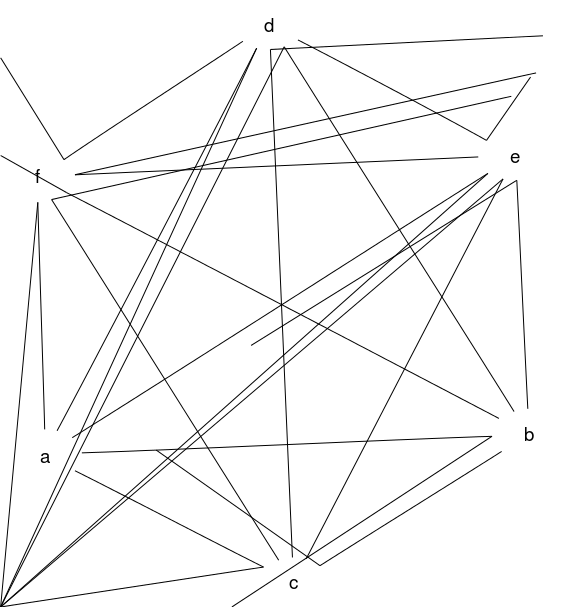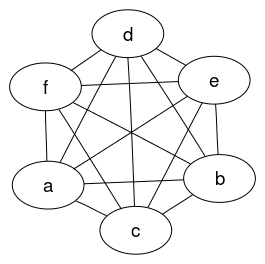# Example 2: K6 complete graph

An undirected complete graph with 6 nodes and 15 edges.

## Graph declaration

In all cases the graph is explicitly declared with ellipse shaped nodes. The dot file edges are declared in alphabetical order as this is the (assumed) default for how Rgraphviz will pass edges internally to graphviz.

 Dot file for graphviz: `````` graph { node [shape=ellipse]; // declare nodes a b c d e f; // declare edges a -- b; a -- f; a -- c; a -- d; a -- e; b -- c; b -- d; b -- e; b -- f; c -- d; c -- e; c -- f; d -- e; d -- f; e -- f; // scale up output to avoid overlap overlap = scale; } `````` R code for Rgraphviz and gridGraphviz: ```# load Rgraphviz library library(Rgraphviz) # nodes and edges nodes <- c("a", "b", "c", "d", "e", "f") edgeList <- list( a = list(edges = c("b", "c", "d", "e", "f")), b = list(edges = c("a", "c", "d", "e", "f")), c = list(edges = c("a", "b", "d", "e", "f")), d = list(edges = c("a", "b", "c", "e", "f")), e = list(edges = c("a", "b", "c", "d", "f")), f = list(edges = c("a", "b", "c", "d", "e"))) # graph object graph <- new("graphNEL", nodes = nodes, edgeL = edgeList, edgemode = "undirected") ```

## Graph rendering:

### gridGraphviz (new)

``````>> neato -Tpng ex2.dot -o ex2.png
`````````# Create Ragraph object
rag <- agopen(graph, "", layoutType="neato",
attrs=list(graph=list(overlap="scale"),
node=list(shape="ellipse")))
```
```#plot
plot(rag)
``````library(gridGraphviz)

# Create Ragraph object
rag <- agopen(graph, "", layoutType="neato",
attrs=list(graph=list(overlap="scale"),
node=list(shape="ellipse")))
```
```#plot
grid.graph(rag)
```
```## Warning: Unsupported node shape; using 'box'
## Warning: Unsupported node shape; using 'box'
## Warning: Unsupported node shape; using 'box'
## Warning: Unsupported node shape; using 'box'
## Warning: Unsupported node shape; using 'box'
## Warning: Unsupported node shape; using 'box'
``````library(gridGraphviz)

# Create Ragraph object
rag <- agopenTrue(graph, "", layoutType="neato",
attrs=list(graph=list(overlap="scale"),
node=list(shape="ellipse")))
```
```#plot
grid.graph(rag)
```## Discussion

gridGraphviz (old) fails to produce ellipse shaped nodes, and produces "extra edges" which extend, in all cases, to the origin.